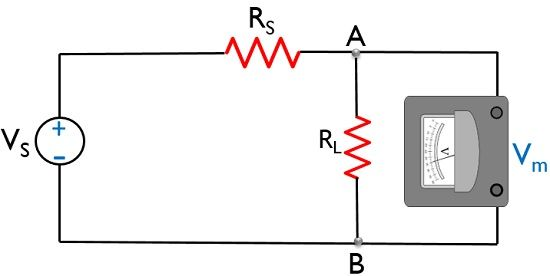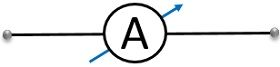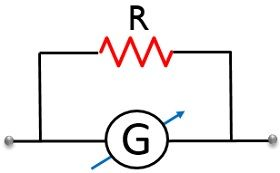# Difference Between Voltmeter and Ammeter

Basically, electricity is measured either in the form of current or voltage. The crucial difference between Voltmeter and Ammeter is that a voltmeter is a device that determines the difference in electric potential between 2 points in the circuit. As against an ammeter determines the amount of electric current flowing through the circuit.

In this article, you will get to know various other differences between voltmeter and ammeter with the help of a comparison chart. But before that put an eye over the contents to be discussed under this article.

## Content: Voltmeter Vs Ammeter

### Comparison Chart

ParameterVoltmeterAmmeter
BasicIt measures voltage.It measures current.
Connected inForms parallel combination with the circuit under measurement. Forms series connection with the circuit under test.
Resistance offeredHighLow
Level of accuracy of resultLowHigh
Measuring unitVolt.Ampere.

### Definition of Voltmeter

Voltmeters are the electrical devices used to determine the voltage across an element or between two specific points in any circuit. Basically, the reading of voltmeter represents the voltage between two points in the circuit.

As we know that voltage across parallelly connected elements is the same. Thus a voltmeter is always parallelly connected with the element across which drop is to be calculated.

Let us consider an ideal voltmeter connected in a circuit having ideal voltage source VS having resistance RSSuppose we have to determine the potential difference between point A and B.

On applying KVL, in the mesh given above,

-VS + 0 Χ RS + Vm = 0

Vm = VS

The figure below represents the symbolic representation of a voltmeter:Also, a voltmeter can be formed by the series combination of a galvanometer with high resistance. This is shown in the figure below:### Definition of Ammeter

An electrical device that is used to determine the current through any circuit is known as an ammeter. More specifically, we can say that the reading of an ammeter shows the amount of current flowing through the circuit.

An ammeter forms a series combination with the circuit under measurement. This is so because, in series combination, the current across the serially connected elements is same.

Thus the ammeter is connected in such a way so as to measure the actual value of current that is flowing through that element.

Consider an ideal ammeter connected in series with an ideal current source IS, with internal resistance RS.It is noteworthy here that RS, in this case, is very high. This is so because the internal resistance of the current source is very high.

On applying KVL to the above circuit, we will get

-IS + 0/RS + Am = 0

Am = IS

The above equation shows that in the ideal case, the overall current supplied to the circuit appears across the load hence is shown by the reading of the ammeter.Resistance in parallel combination with a galvanometer acts as an ammeter, in order to determine the current flowing through the circuit as shown below:## Key Differences Between Voltmeter and Ammeter

1. A voltmeter shows the reading of potential difference while ammeter determines the electric current.
2. A voltmeter is parallelly connected along with the points within which the potential difference is to be calculated. While a serial connection of ammeter is formed with the element through which the flowing current is to be calculated.
3. Practically the resistance offered by the voltmeter is high. However, the resistance offered by ammeter is low.
4. The accuracy of the result is comparatively low in case of voltmeter than an ammeter.
5. As voltmeter measures voltage thus its measuring unit is Volt. While ammeter measures current thus its measuring unit is Ampere.
6. In an ideal case, voltmeter offers infinite resistance whereas ammeter provides 0 resistance.

### Conclusion

So, from the above discussion, it can be concluded that both of these electrical devices are placed in the circuit for their respective application. However, a multimeter is the instrument that can be used to function both as voltmeter and ammeter.